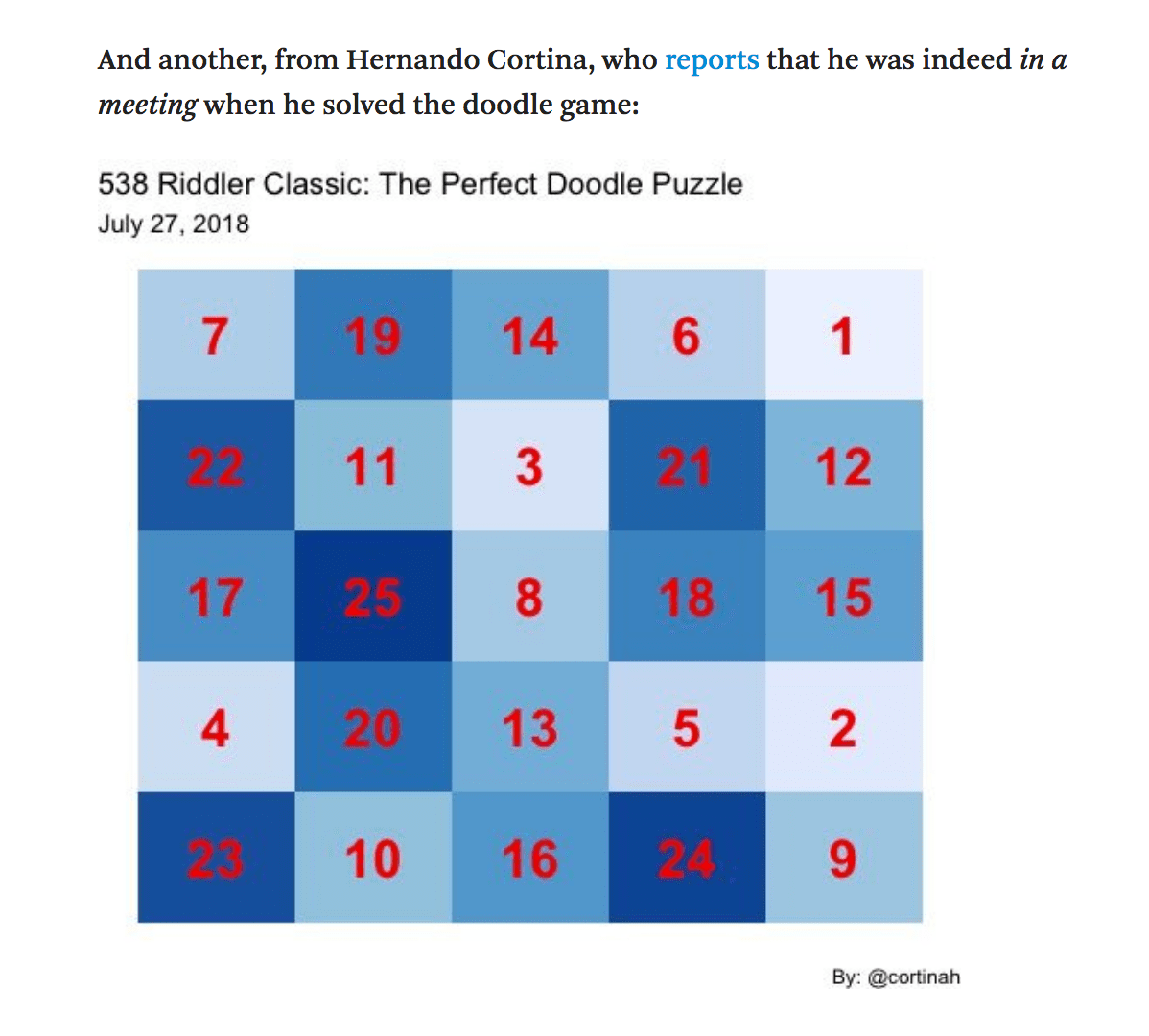Want to share your content on R-bloggers? click here if you have a blog, or here if you don't.

## Apologia

It’s been quite a while since my last post and I regret that. I’ve been rather busy, mainly with this. Now that’s succesfully out of the way I have a little more time to write a few words and post a little R code here. Despite the lack of blogging, I always seem to find the time for a little FiveThirtyEight Riddler puzzle. I was thrilled when a few weeks ago Riddler editor Oliver Roeder thought my simulation of dystopic row house collapse times was beautiful. Here’s the viz:

Seldom do people use that precise word regarding the finance-oriented kind of charts I usually make, and it was gratifying to hear that since I did focus on the aesthetics of that visualization. On this post I write about a more recent Riddler puzzle, this one is a little more code heavy, but I did manage to slip in a little colorbrewer for a splash of color in the final result.

## On the July 27, 2018 FiveThirtyEight Riddler Classic

This Riddler Classic, was about filling a 5×5 grid with all numbers from 1 to 25, subject to the provisos that from your chosen starting cell you may: 1) move exactly 3 cells horizontally or vertically, or exactly 2 cells diagonally; 2) you are not allowed to visit any cell twice; and 3) you may not step outside the grid. You win if you fill in all the cells.

My solution was based on selecting an initial cell at random, checking which of the eight possible moves are valid, and randomly selecting among those. This process is repeated until there are no more valid moves and the player has lost the game, or until all cells have been selected once and the player has won the game. The R code below is broken into three functions that perform the checking for valid moves, returning the next position, and updating and checking the state of the board. The search() function then repeats this process until a solution is found. Thanks for reading and please reach out with any comments.

### Function to check valid moves given the state of the board and current position

```moves <- function(board, pos) {
pos_moves <- rep(FALSE,8)
names(pos_moves) <- 1:8

if ((pos+3) < 6) (if (board[ pos+3,pos ]==F) pos_moves <- T)
if ((pos-3) > 0) (if (board[ pos-3,pos ]==F) pos_moves <- T)
if ((pos+3) < 6) (if (board[ pos,pos+3 ]==F) pos_moves <- T)
if ((pos-3) > 0) (if (board[ pos,pos-3 ]==F) pos_moves <- T)
if ((pos+2) < 6  & (pos+2) < 6) (if (board[ pos+2,pos+2 ]==F) pos_moves <- T)
if ((pos+2) < 6  & (pos-2) > 0) (if (board[ pos+2,pos-2 ]==F) pos_moves <- T)
if ((pos-2) > 0  & (pos-2) > 0) (if (board[ pos-2,pos-2 ]==F) pos_moves <- T)
if ((pos-2) > 0  & (pos+2) < 6) (if (board[ pos-2,pos+2 ]==F) pos_moves <- T)

pos_moves
}```

### Function that returns next position given current position and selected move

```next_pos <- function(pos, path) {

pos <- switch(path, c(pos+3, pos), c(pos-3, pos),
c(pos, pos+3), c(pos,pos-3),
c(pos+2,pos+2), c(pos+2,pos-2),
c(pos-2,pos-2), c(pos-2,pos+2) )
pos
}```

### Main game function

```game <- function() {

# Set-up board and initial location
board <- matrix(F,ncol=5,nrow=5)
current <- sample(1:5,2,replace=T)

board[current,current] <- T
i <- 1
pos_hist <- vector("list", 25)
pos_hist[[i]] <- current

repeat {
possible <- moves(board, current)
if (all(possible==F)) {ret <- 1; break}
possible <- possible[possible==T]
path <- sample(possible,1)
current <- next_pos(current, as.numeric(names(path)))
board[current,current] <- T
i <- i+1
pos_hist[[i]] <- current
if (all(board==T)) {ret <- 0; break}
}

return(list(ret,pos_hist))
}```

### Keep searching until a solution is found

```search <- function(){

repeat{
t <- game()
if (t[]==0) break }
t[]
}```

### Solve and plot

```library(ggplot2)
t <- search()

path <- as.data.frame(matrix(unlist(t), ncol=2, byrow = T))
path\$step<-1:25
colnames(path)<-'x'
colnames(path)<-'y'

ggplot(path,aes(x,y,fill=step)) +geom_tile() +scale_fill_distiller(direction = 1) +
geom_text(aes(label=step),size=8,color='red',fontface='bold') +theme_void() +
theme(legend.position='none') +labs(title='538 Riddler Classic: The Perfect Doodle Puzzle',
subtitle='July 27, 2018',caption='By: @cortinah') +coord_fixed()```I was fortunate to once again, together with Luke Benz, receive a mention by Ollie last week.

### Thanks again, Ollie! P.S.: That wasn’t a work meeting of course…Note: My submitted answer is different from the one generated from the above code due to the randomness in the search process. Should have set.seed() in my original submission to make it reproducible.

## Epilogue

Luke Benz clearly loves a good puzzle and I noticed he posted a series of solutions not just for the 5x5 puzzle, but also for the 6x6, 7x7, and 8x8 versions. In the spirit of friendly competition I took a shot at the 9x9 variation. All that is required in the above code is to change the board matrix ncol and nrow to be 9x9, change the bounds checks in moves() to be 10 rather than 6, and change the size of the pos_hist list to 81. Of course, the complexity of the solution increases quadratically with the grid’s length. The code took a little while to find a solution, but when I got back from lunch, there it was. Much rejoicing ensued.